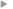## Methods for Computing the Boiling Temperature of Water at Varying Pressures

#### Miller, Sam

Année de publication
2017
Résumé
Boiling is an extreme form of evaporation that occurs when the saturation vapor pressure is equal to the total atmospheric pressure. This paper computes boiling temperature by four different methods and compares the results to data provided in the CRC Handbook of Physics and Chemistry. The first method utilizes a constant value for the latent heat of vaporization (lυ) in the Clausius–Clapeyron equation, and the second method uses a previously published temperature-dependent linear function for lυ. A third method is suggested that begins by deriving a new second-order function for lυ, and then applies this function in an error-reduction loop to determine boiling temperature as a function of pressure. This method for computing boiling temperature shows a mean bias of 0.31°C between mean sea level pressure and 100 hPa and a mean error of less than a tenth of a percent, which is a significant improvement over both of the first two methods. The fourth method uses a fifth-order polynomial, eliminating lυ and making pressure the independent variable, which is used to compute boiling temperature with a mean bias of 0.25°C and a mean error of 0.09% for pressures between mean sea level pressure and 100 hPa.Accès à la notice sur le site du portail documentaire de Météo-FranceListe complète des notices publiques